# Python Variables & DataTypes

## Variables in python

In general, the variables are the names that hold a data stored in the memory and it should have an unique name known as identifier.

## Declaring and assigning value to a variable

Python is a dynamically typed language.
In Python you do not need to declare a variable type, because the types are determined automatically at runtime (this is know an dynamically typed) when we assign a value to it.
The data what we assign to a variable takes the form of objects. i.e. either built-in objects that Python provides, or objects we create using Python classes.
Objects are just pieces of memory, with values (and sets of associated operations)
Let us check the following example.

``````x = 5
name = "Python"
salary =  60.50
``````

Here x, name and salary are the variable names on the left side of "=" on each statement and on the right side all of its values that are assigned.
Note that we have not pre-declared the variable names and its type before assigning values (generally required in languages like C, C++ , Java).

5, "Python" and 60.50 are nothing but the literals that creates the objects of a particular type. So "x" holds integer type data, "name" -> string and "salary" -> float type of data with 5, "Python" and 60.50 values respectively within it

Python does this process of determining type automatically at the run time, when we assigned data objects or values (5, "Python" and 60.50 ) which are known as built-in object types or standard data types in Python.

Note *

If a variable is not “defined” (assigned a value), trying to use it will give you an error:

``````print(N) # N is not defined
``````
Traceback (most recent call last):
File "D:/pythoneasy/variables.py", line 1, in <module> print(N) NameError: name 'N' is not defined

### How does Python process a variable

Everything we process in Python programs is a kind of object.
Object is a distinct piece of memory.
Each time you generate a new value in your program by running an expression like above, Python creates a new object ("5" in this case) to represent that value for you
When we write the expression `x = 5`, Python interpreter does the following steps

1. It creates an object to represent 5 in the memory (the type determined automatically which is integer here )
2. It creates a variable name x
3. It creates links the variable x to object 5 which known as reference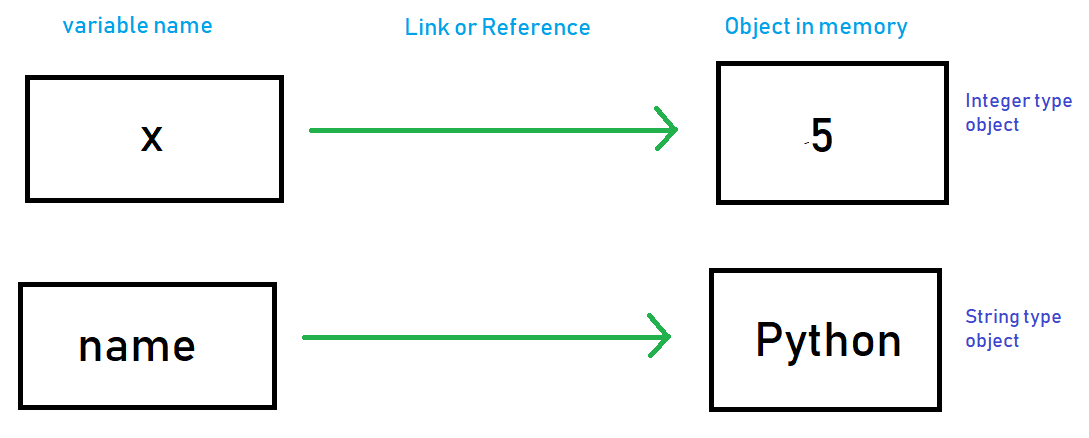### Changing value of variables

Let us see the below example

``````x = 5   # It's an integer
x = "amazon" # It's a string
x = 40.25   # It's a float
``````

We assign 5 to "X" it becomes integer type (it refers to an integer type object 5 in actual).
When we reassign "amazon" which a string type of object to "x" it becomes a string type. Eventually in this step Python freed the memory that object "5" holds. This is a part of automatic garbage collection by python
The we reassign 40.25 a float value to "x" and it becomes float type and Python frees the memory that "amazon" holds.

The type changes as we keep on assigning different type of object to it and the variable keep changing its reference to that object in memory
Therefore the names or variables names does keep the type but the type lives with objects ( 5, "amazon", 40.25), so to whomever these gets assigned the names become that particular type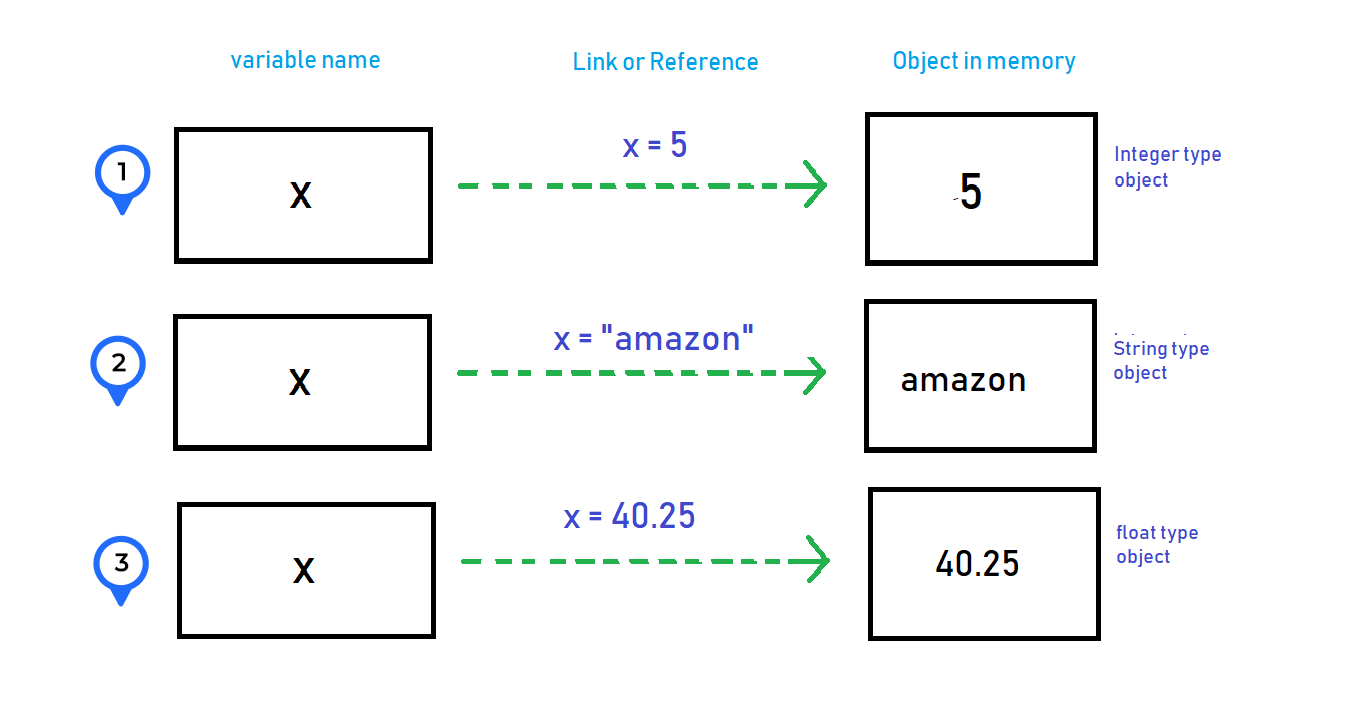### Shared Reference

Let us see this example

``````x = 5
y = x
``````

We assign 5 to "x", so it refers/links to an object "5" in memory.
When we assign "y" to "x" both the "x" and "y" refers to same object of "5" (Check the image below) which is known as shared reference.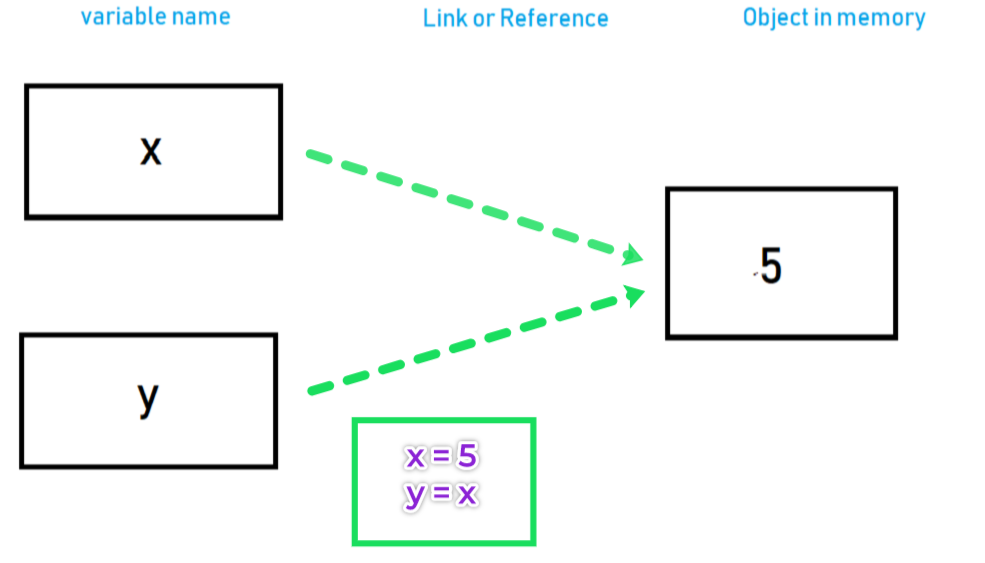``````x = 5
y = x
x = "amazon"
``````

`x = "amazon"` - here we reassign a new string value to "x", so a new object with string "amazon" is created and then Python sets "x" to refer it
Now this time "y" value is not changed ans it still refers to the old integer object "5" in the memory (Check the image below)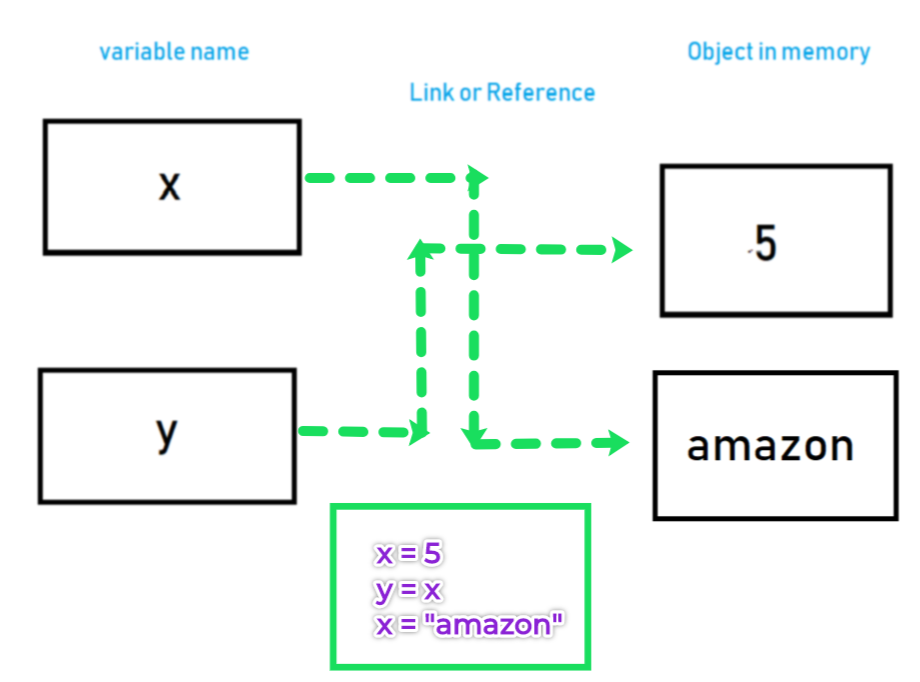### Multiple Assignment

We can assign multiple values to multiple variables in a single line.
Example:

``````x, y, z = 10, 20, "amazon"
print(x) # 10
print(y) # 20
print(z) # "amazon"
``````
10
20
amazon

We can assign same value to multiple variables at a time in single line
Example:

``````x = y = z = 10
print(x)
print(y)
print(z)
``````
10
10
10

## Standard or built-in data types with Literals

Python has various built-in data or object types.
The following is the Python's built in data types and their respective literals.
Literals - are the expressions that generates the objects

The Python data types are divided into 2 categories i.e. mutable and immutable types.
mutable are those objects once assigned can be altered later
immutable data type objects can not be changed once assigned but rather return new objects when attempting to update.

• int
• float
• decimal
• complex
• bool
• string
• tuple
• range
• frozenset
• bytes

#### The following are some mutable objects

• list
• dict
• set
• bytearray
• user-defined classes (unless specifically made immutable)

In this section we will only discuss few of them (listed below)

Data types Literals or Expression to create objects
Number 1234, 3.1415, 5+7j, 0b101
String "pythoneasy", 'pythoneasy', "python's easy" , 'python"s easy', b'c\x02d', u'e\xc4sy'
Lists , [5, "pythoneasy", 4.5] , list('easy')
Tuple (5, "pythoneasy", 4.5), tuple('easy')
Dictionary {"name": 'pythoneasy', 'year': 2004}, dict(name="pythoneasy")
Set set('pythoneasy'), {'e', 'a', 's','y'}

### Numbers

In Python number data type objects store numeric data
Python supports the following numeric data types

• int (signed integers, binary, octal and hexadecimal) - whole number, positive or negative, without decimals, of unlimited length
• float (floating point values ) - positive or negative, containing one or more decimals
• complex (complex numbers) - written with a "j" as the imaginary part

Numeric types are created by assigning numeric values to the variables
Examples

``````x = 10  # int
y = 7.5 # float
z = 2j  # complex
``````

#### The type() built in function

Python has a set of built-in functions
The type() built in function helps to determine the type of the object.

``````print(type(x))
print(type(y))
print(type(z))
``````
<class 'int'>
<class 'float'>
<class 'complex'>

More Examples

``````# Decimal literals
x = 400
z = 2652565188198713537826762234234234 # very long numbers are integers
k = -30 # negative numbers

# Binary literals
bin1 = 0b1101   # starts with 0b (zero b)

# Octal literals
oct1 = 0o234    # starts with 0o (zero and "o" )

hex1 = 0x14b     # starts with 0x (zero and ex )

#   Floats
float_literal1 = 34.12
float_literal_neg = -46.09
#   Float can also be scientific numbers with an "e" to indicate the power of 10.
float_literal2 = 65e3
float_literal3 = 23E4

#   Complex Number
c = 5.23j

print(x, type(x))
print(bin1, type(bin1))
print(oct1, type(oct1))
print(hex1, type(hex1))
print(float_literal1, type(float_literal1))
print(c, type(c))
``````
400 <class 'int'> 13 <class 'int'> 156 <class'int'> 331 <class 'int'> 34.12 <class 'float'> 5.23j <class 'complex'>

The Binary, Octal and Hexadecimal literals are converted to integer type when printed

#### Numeric Operations

The operators +, -, * and / can be used to do various operations on the numbers

``````>>> 3 + 3
6
>>> 60 - 5*6
30
>>> (60 - 5*6) / 4
7.5
>>> 18 / 5  # division always returns a floating point number
3.6

>>> 17 / 3  # classic division returns a float
5.666666666666667
>>>
>>> 17 // 3  # floor division discards the fractional part
5
>>> 17 % 3  # the % operator returns the remainder of the division
2
>>> 5 * 3 + 2  # result * divisor + remainder
17

>>> 5 ** 2  # 5 squared
25
>>> 2 ** 7  # 2 to the power of 7
128
``````

### Strings

A string literal is simply a list of characters (textual data) in sequence surrounded by quotes.
A character can be anything like numbers, backslash or letters and can also have space.
For example,`"easy"`is a string.
It is a four character long with each characters in sequence `"e", "a", "s", "y"`
We can assign the same string to a variable as follows

``````mystring = "easy" # create a 4 character string objects and assign it to name "mystring"
``````

mystring is the variable name to which the string object is assigned.
There are fours ways you can declare a string literal (or create a string object) in Python i.e. single quotes ('), double quotes ("), triple double quotes (""") and triple single quotes (''')
Example:

``````string1 = 'It is a single quoted string'
string2 = "It is a double quoted string"
string3 = """It is a triple double quoted string"""
string4 = '''It is a triple single quoted string'''
multiline_string1 = """
This is a
sting in multiple lines
"""
multiline_string2 = '''
This is a
sting in multiple lines
'''
unicode_string = u'e\xc4sy'
print(type(string1))
print(type(unicode_string))
``````

<class 'str'>
<class 'str'>

type() - is a built-in function which returns the type to which the object belongs. Refer to https://docs.python.org/3.7/library/functions.html#type
multilinestring1 and multilinestring2 are multi-line string literals. We can write multiple line texts/string using triple double quotes (""") and triple single quotes (''')
unicodestring is unicode string literal.

#### Indexing

The characters are stored in order or sequence in the memory with a particular position assigned to each of the character.
The positions or the offsets are known as index.
Example:

``````mystring = "easy" # create a 4 character string objects and assign it to name "mystring"
``````
When we assign the value "easy" to mystring the value get stored in memory as follows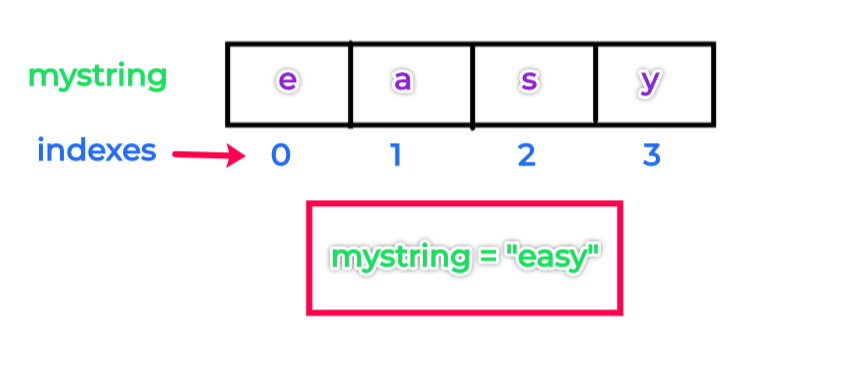The first item or character "e" is at index 0 and the second item "a" is at index 1 ans so on
Items or characters from the string can be retrieved by using the name ( e.g. mystring ) and these indexes. The process is called indexing

As "e" is at index 0 we can retrieve and print "e" using name mystring as follows

``````print(mystring)
``````

e
To retrieve "a" and others

``````print(mystring)
print(mystring)
print(mystring)
``````

a
s
y

#### Negative Indexing

we can also index backward. The above positive indexes from 0, 1, 2 and so on -count form left to right
The negative index count from right to left starting with -1 e.g. -1, -2, -3.
So from the previous example `mystring = "easy"`,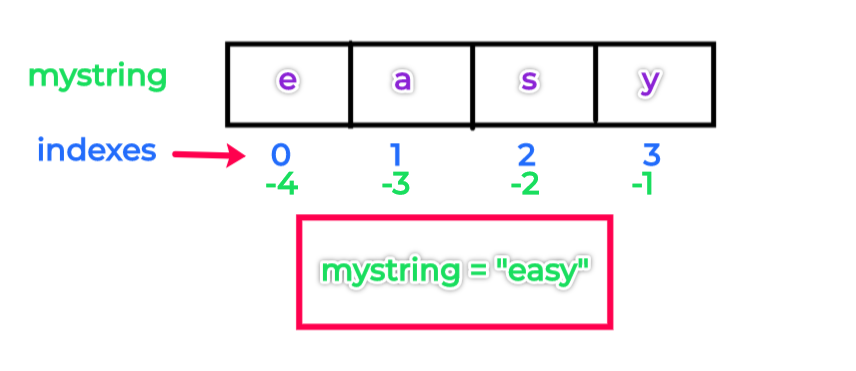if we want to retrieve "a" using mystring and negative index we can write

``````print(mystring[-3])  # is same as mystring
``````

a So the value at 1 is same as -3 and 0 is as index -4

#### Slicing

We can also extract a portion of a string using indexes know as slicing.
The syntax is

``````variable_name[I:J] # Gives everything in variable_name from I to J, but inot including J
``````

Example:

``````print(mystring[1:3])
``````

as
So it extracts the string from offset/index 1 to 2 (Not including 3)

### Lists

Python lists are ordered collections of arbitrarily typed objects, can be written as a list of comma-separated values (items) between square brackets. Lists are mutable type that means they can be changed after assigned.

Example :
``````mylist = ["pythoneasy", 2016, "english"]
print(type(mylist))
``````
<class 'list'>

#### Indexing

Like strings, lists can be indexed and sliced.
As list is a sequence data type, the items or objects are stored in the memory in the sequence with index starting from 0 (as shown in the below image)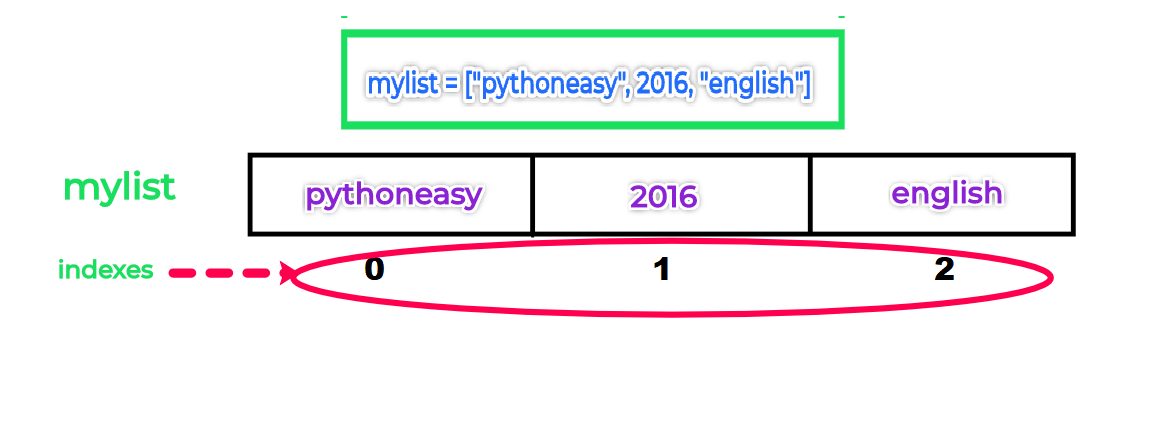so each element is referred to a index.
In the above example "pythoneasy" is linked to positional index 0 and 2016 to 1 and "english" to 2
We can access these with reference mylist, mylist and mylist

``````mylist = ["pythoneasy", 2016, "english"]
print(mylist) # prints "pythoneasy"
print(mylist) # prints 2016
print(mylist) # prints "english"
``````
pythoneasy
2016
english

Lists also support operations like concatenation:

``````squares = [1, 4, 9, 16, 25]
print(squares + [36, 49, 64, 81, 100])
``````
[1, 4, 9, 16, 25, 36, 49, 64, 81, 100]

Unlike strings, which are immutable, lists are a mutable type, i.e. it is possible to change their content using index

``````cubes = [1, 8, 27, 65, 125]
cubes = 64  # replace the value at index
print(cubes)``````
[1, 8, 27, 64, 125]

#### Negative Indexing

When we create a list, the negative index will be created from right to left starting from -1 (as shown in the below image)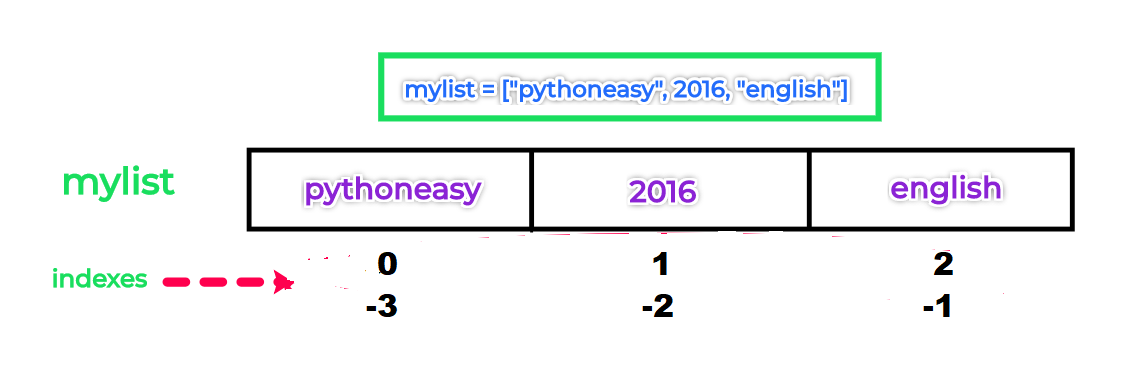``````mylist = ["pythoneasy", 2016, "english"]
``````

In the above example mylist[-1] provides the last value always which is "english"
And the value of mylist[-3] is same as mylist and mylist[-2] as mylist

``````print(mylist[-1])
``````
english

#### Slicing

We can also extract a range of a items from a list using indexes know as slicing.
The syntax is

``````variable_name[I:J] # Gives everything in variable_name from I to J, but inot including J
``````

Example:

``````mylist = ["pythoneasy", 2016, "english", 2017, 2018]
print(mylist[1:4])
``````

[2016, 'english', 2017]

### Tuples

Tuples in python are similar as Lists. But tuples are immutable where as lists are mutable. That means you can not change the values of tuple once assigned
Tuples can contain any type of data like lists

A tuple consists of a number of values separated by commas

``````t = 12345, 54321, 'hello!'
print(t) # indexing like lists``````
12345

Tuples can be created by using parentheses ()

``````t = (12345, 54321, 'hello!')
print(t)``````
12345

Tuples are immutable
``````t = 12345, 54321, 'hello!'
t = 88888``````
Traceback (most recent call last): File "D:/pythoneasy/test.py", line 2, in <module> t = 88888 TypeError: 'tuple' object does not support item assignment

### Dictionaries

A dictionary in Python is a collection of unordered series of “key: value” pairs

Unlike sequences, which are indexed by a range of numbers, dictionaries are indexed by keys
Dictionaries are coded in curly braces using key value pairs. The keys should be unique and hashable (immutable) types

``````d = {"website": "pythoneasy", "year": 2016} # website, year are the keys and pythoneasy, 2016 are the values
print(d["website"]) # Indexing can be done using keys``````
pythoneasy

Using assignment operator and the dictionary name we can update an existing value or add new value with a key

``````d = {"website": "pythoneasy", "year": 2016}
# Change the value of key "website"
d["website"] = "python.org"
print(d) # prints {'website': 'python.org', 'year': 2016}

# Add a new value "english" with key "language"
d["language"] = "english"
print(d) # prints {'website': 'python.org', 'year': 2016, 'language': 'english'}
``````

{'website': 'python.org', 'year': 2016}
{'website':'python.org', 'year': 2016, 'language': 'english'}

The value for key "website" is updated and a new value for the key "language" is created as the key is not available in d

### Sets

A set is an unordered collection with no duplicate elements.A set is mutable data type

Basic uses include membership testing and eliminating duplicate entries. Set objects also support mathematical operations like union, intersection, difference, and symmetric difference

To create a set object we need to use curly braces {}

``````myset = {"pythoneasy", 2016, "english"}
print(myset) # prints {2016, 'pythoneasy', 'english'}
``````
{2016, 'english', 'pythoneasy'}